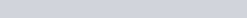# Numbers Set Analogy Questions with Solution

Q.21: Select the option in which the numbers are related in the same way as are the numbers of the following set.
(3, 3, 11)

a) (4, 5, 18)
b) (5, 8, 26)
c) (7, 11, 35)
d) (6, 15, 47)

Ans : c) (7, 11, 35)
Solution:
(3+3) x2 -1 =11
Similarly
(7+11) x2 -1 =35

Q.22: Select the option in which the numbers share the same relationship as that shared by the numbers in the given set.
(7, 4, 33)

a) (9, 5, 56)
b) (7, 4, 71)
c) (8, 5, 40)
d) (11, 8, 175)

Ans : a) (9, 5, 56)
Solution:
72-42=49-16=33
Similarly
92-52=81-25=56

Q.23: Select the option in which the numbers are related in the same way as are the numbers of the following set.
32 : 63 : 123

a) 26 : 48 : 92
b) 26 : 51 : 100
c) 35 : 72 : 149
d) 33 : 66 : 130

a) 26 : 48 : 92
Solution:
32×2=64, 64-(3-2) =63, 63×2=126, 126-(6-3)=123
Similarly
26×2=52, 52-(6-2)=48, 48×2=96, 96-(8-4) =92

Q.24: Select the option in which the numbers are related in the same way as are the numbers of the following set.
(92, 85, 82)

a) (28, 23, 21)
b) (71, 65, 62)
c) (83, 78, 77)
d) (69, 66, 62)

c) (83, 78, 77)
Solution:
92+85=174, 174/2 =87, 87-2=85
Similarly
83+77=160, 160/2=80, 80-2=78

Q.25: Select the set in which the numbers are related in the same way as are the numbers of the following set.
(697, 13, 866)

a) (832, 8, 887)
b) (747, 25, 1423)
c) (651, 16, 940)
d) (541, 14, 737)

d) (541, 14, 737)
Solution:
697+132=697+169=866
Similarly
541+142=541+196=737

Q.26: Select the option in which the numbers are related in the same way as are the numbers of the following set.
(25, 17, 170)

a) (23, 18, 421)
b) (49, 20, 140)
c) (24, 60, 240)
d) (36, 12, 144)

d) (36, 12, 144)
Solution:$\sqrt{25} =5, 5\times2 =10, 10\times17=170$
Similarly$\sqrt{36} =6, 6\times2 =12, 12\times12=144$

Q.27: Select the option in which the numbers are related in the same way as are the numbers of the following set.
(16, 60, 240)

a) (16, 38, 260)
b) (14, 42, 196)
c) (18, 56, 252)
d) (20, 12, 121)

c) (18, 56, 252)
Solution:
240/16=15, 15×4 =60
Similarly
252/18 = 14, 14×4=56

Q.28: Select the set in which the numbers are related in the same way as are the numbers of the following set.
(13, 37, 77)

a) (15, 43, 83)
b) (12, 38, 79)
c) (14, 40, 83)
d) (17, 53, 109)

c) (14, 40, 83)
Solution:
13×3-2=37, 13×6-1=77
Similarly
14×3-2=40, 14×6-1=83

Q.29: Select the option in which the numbers share the same relationship as that shared by the numbers of the given set.
(277, 14, 9)

a) (364, 11, 12)
b) (123, 17, 8)
c) (313, 12, 13)
d) (198, 15, 8)

c) (313, 12, 13)
Solution:
142+92=196+81=177
Similarly
132+122=169+144=313

Q.30: Select the set in which the numbers are related in the same way as are the numbers of the following set.
(4, 140, 6)

a) (9, 396, 4)
b) (2, 121, 6)
c) (5, 234, 7)
d) (3, 269, 8)